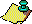Contents Next: Lyapunov Exponents Up: Classical Results Interpreted Previous: Periodic and Fixed

Of course, non-quadratic irrationals have continued fraction expansions, too. By Lagrange's theorem, these continued fractions are aperiodic, and hence the orbit of these initial points under the Gauss map is aperiodic. Note that most numbers in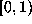are thus aperiodic. We examine some beautiful examples, beginning with one due to Euler:
1. e (the base of the natural logarithms) has an aperiodic continued fraction expansion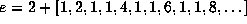. The elements of the orbit of this initial point are always of the form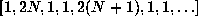,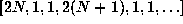, or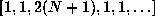, which tend to 1, 0, and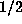, respectively. Thus the-limit set of this orbit is the set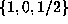, which, unlike the- limit sets of continuous maps, is not invariant under the Gauss map since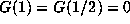so G applied to this set simply gives 0. In other words, we have an asymptotically periodic orbit which is not asymptotic to a real orbit of the map. This cannot happen for a discrete dynamical system with a continuous map.
2. Stark . If x is the positive root of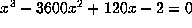, then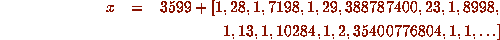which has very large entries placed irregularly throughout. This intermittency is a typical feature of a chaotic system .

3. Lambert, 1770--- cf . The continued fraction foris not known, in the sense that no pattern has been identified. It begins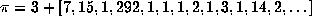and some 17,000,000 elements of this continued fraction have been computed by Gosper (see ). There are many open questions about this continued fraction---for example, it is not known if the elements of the continued fraction are bounded.Contents Next: Lyapunov Exponents Up: Classical Results Interpreted Previous: Periodic and Fixed